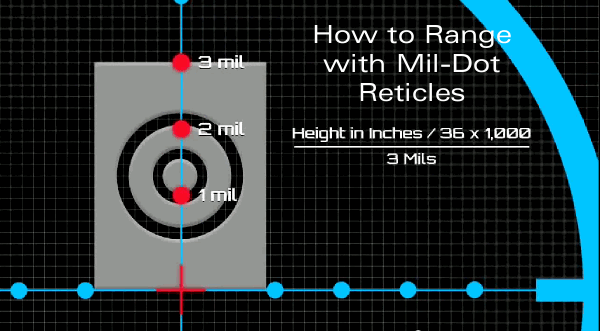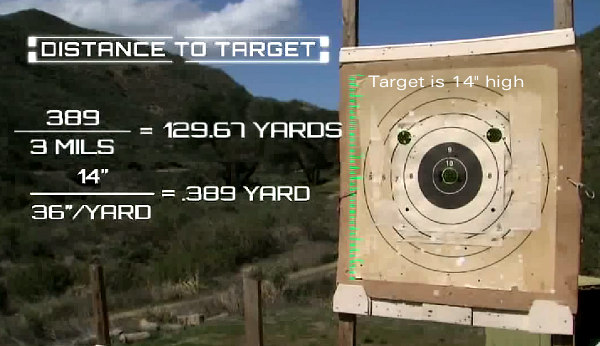July 26th, 2021

## How to Range Targets with MIL-Reticle OpticsMIL-system scopes are popular with tactical shooters. One advantage of MIL scopes is that the mil-dot divisions in the reticle can be used to estimate range to a target. If you know the actual size of a target, you can calculate the distance to the target relatively easily with a mil-based ranging reticle. Watch this helpful NRA video to see how this is done:

Milliradian Definition and Yardage Ranging Formula
“MIL” or “Milrad” is short-hand for Milliradian, a unit of angular measurement. The subtension of 1 mil equals 3.6 inches at 100 yards or 36 inches at 1,000 yards. (In metric units, 1 mil equals 10 centimeters at 100 meters or 1 meter at 1,000 meters.) Knowing this subtension and knowing the size of the target (or a reference object near the target) allows the distance to the target to be estimated with considerable accuracy. The formula used to calculate range (in yards) based on MIL measurement is:

Height of Target in inches (divided by 36) x 1000, divided by the number of mils.For example, if a 14″ tall target spans 3 mils from top to bottom, the distance is 129.67 yards calculated as follows: 14/36 x 1000 = 389, then divided by 3 = 129.67. You can also use a different conversion to find distance in meters.

Can You Estimate Range with an MOA-Marked Reticle? Yes You Can…
Reader Josh offers this handy advice: “It worth noting that the ability to measure range is not unique to mil-based systems. A MIL is just another unit for measuring angles, and any angular measurement will work. Considering that just about everybody knows that 1 MOA is about an inch per hundred yards, similar formulae can be developed for ranging with MOA marks. The advantage with mils is the precise relationship between units — the MOA-inch measurement is imprecise (being off by 0.047″) — so in principle MILs are a better unit”.